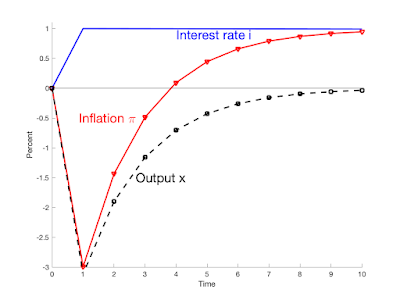# The Grumpy Economist: Fiscal Histories

Fiscal Histories  is a new paper, a second try at an essay on fiscal theory for the Journal of Economic Perspectives.

The basic concept is to explain the fiscal theory of the price level with no equations by applying it, by seeing how it might be able to explain and interpret a wide variety of historical episodes. Abstract:

The fiscal theory states that the price level adjusts so that the real value of government debt equals the present value of real primary surpluses. Monetary policy remains important. The central bank can set an interest rate target, which determines expected inflation, and news about the present value of surpluses drives unexpected inflation. I exposit fiscal theory by offering an interpretation of historical episodes, including the gold standard, currency pegs, the ends of hyperinflations, the success of inflation targets, the rise and fall of inflation in the 1970s and 1980s, the long quiet zero bound of the 2010s, and the 2021-2022 inflation. Going forward, fiscal theory warns that inflation will have to be tamed by coordinated monetary and fiscal policy.

I thank Erik Hurst and Tim Taylor for the concept. Most of the stories are summarized from the Fiscal Theory of the Price Level, but pulling them out, putting them in one place and simplifying them is a great idea. The key idea

I think through how fiscal theory can account for important episodes. A first purpose is expositional: by this method we can understand how fiscal theory works, and what elaborations it might need. A second purpose is more serious: analyzing episodes is the crucial way we evaluate all macroeconomic models.

I mostly tell plausible stories, rather than summarize well-worked out and published economic history or quantitative analysis. Fiscal theory is new, and that work is just beginning. But stories rightly come first. Formal analysis always builds on plausible stories. Moreover, that there are such plausible stories, that it provides a framework that can possibly account for history as MV=PY and IS-LM do, is news, since many people opine that fiscal theory can be quickly dismissed by well-known episodes. I also hope to inspire detailed analysis.

The paper also shows the results of a nice model, and sneaks the model in a footnote, that gets at the basics of fiscal theory with sticky prices more simply than I did in FTPL. The graph:Figure 1: Responses of inflation and output in a simple fiscal-theory sticky-price model. Top: 1% deficit shock with constant interest rate. Bottom: Interest rate shock with constant surpluses

You can think through a lot of fiscal theory with these graphs. The first graph is a fiscal shock with no change in interest rate, which is roughly what I think we saw in 2021. It leads to protracted inflation, and devalues outstanding bonds with a period of negative real interest rates. The second graph is an interest rate rise with no change in surpluses. It gives a temporary inflation decline. Reality combines the two graphs. The Fed’s rate rises will add the second graph on the first, which will help. A bit. For a while.

Yes, repackaging the same ideas in a lot of different ways. I hope to find one that works, or at least to offer different packages for different tastes.

I’m not sure of the title yet. Fiscal Fables? Fiscal Bedtime Stories? ; )

The model is \begin{aligned}x_{t} & =E_{t}x_{t+1}-0.5(i_{t}-E_{t}\pi_{t+1})\\\pi_{t} & = E_{t}\pi_{t+1}+0.5 x_{t}\\i_{t} & = i_{t-1} + \varepsilon_{i,t} \\ \rho v_{t+1} & =v_{t}+r_{t+1}^{n}-\pi_{t+1}-\tilde{s}_{t+1}\\E_{t}r_{t+1}^{n} & =i_{t}\\r_{t+1}^{n} & =0.9q_{t+1}-q_{t}\end{aligned}where $$x$$ = output gap, $$i$$=interest rate, $$\pi$$=inflation, $$v$$= real value of government debt, $$r^n$$=nominal return on government debt,   $$\tilde{s}$$ = real primary surplus scaled by value of debt, $$q$$= log price of government debt. Debt has a geometric maturity structure with coefficient 0.9.